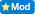I DARE YOU TO ANSWER THIS QUESTION ( PART 5 )

IF  3+2=10

3+4=28

7+5=60

THEN  7+9=____???

I DARE YOU TO ANSWER IT AND TELL ME HOW DID YOU KNOW THE ANSWER !

0  Views: 494 Answers: 4 Posted: 10 years ago
ColleenApparently no one wants to play. Part 4 from a month ago never even got answered. Is this your unique way of getting your homework finished?
millie111

ROTFL!!...Nice try though...you have to give him/her credit for being SO cheeky!!!;-))

You figure out how to get there since is your home work anyway.

PS Your question is part 4 rather than 5.

You're taking the fun out of this site. I don't want to do your homework either............

Good point at my age I'm taking no chances , also at my age i dont need to know unless it involves cash..

IF 3+2=10  ( 10 is 2x 3 + 2)

3+4=28  (28 is 4x  3 + 4)

7+5=60  (60 is 5x 7 + 5)

THEN 7+9=____???  7 + 9 = 16 x 9 = 144

You have multiple the sum of each equation by the second addend.

### Top contributors in Uncategorized category

ROMOS
Karma: 1101K

Colleen
Karma: 953K

country bumpkin
Karma: 838K

Benthere